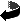# The smallest Dirac eigenvalue in a spin-conformal class and cmc-immersions by Bernd Ammann

The smallest Dirac eigenvalue in a spin-conformal class and cmc-immersions (.ps,.pdf)
Comm. Anal. Geom. 17 (2009), 429-479.

### Abstract

Let us fix a conformal class [g_0] and a spin structure σ on a compact manifold M. For any g\in [g_0], let λ+1(g) be the smallest positive eigenvalue of the Dirac operator D on (M,g,\si). In a previous paper we have shown that \lambda(M,g,\si):=\inf_{g\in [g_0]} λ+1(g) vol(M,g)^{1/n}>0.
In the present article, we enlarge the conformal class by certain singular metrics. We will show that if $\lambda(M,g,\si)<\lambda(S^n)$, then the infimum is attained on the enlarged conformal class. For proving this, we have to solve a system of semi-linear partial differential equations involving a nonlinearity with critical exponent: $D\phi= \la |\phi|^{2/(n-1)}\phi.$
The solution of this problem has many analogies to the solution of the Yamabe problem. However, our reasoning is more involved than in the Yamabe problem as the spectrum of the Dirac operator is not bounded from below. The solution may have a nonempty zero set because a maximum principle is not available.

Using the Weierstraß representation, the solution of this equation in dimension 2 provides a tool for the construction of new constant mean curvature surfaces.

### Typo in the published version

In the second last line at the end of section 4 the term k-1/3 should be replaced by k-1/2.

### Warning for citations

Unfortuately the journal has changed the numbering of theorems, propositions, and equations in the final version in such a way that after equation (5.4) we have Theorem 5.2 and later Proposition 5.1. As I do not want to adapt the preprint version to this numbering, the numbering in the preprint version and the published version differ.

### A further comment about regularity

(All numbers correspond to the preprint version linked above)
In Theorem 5.1 we claim that equation (5.2) holds in weakly, and φ is in Lq for the critical q. It is natural to ask whether φ has higher regularity, e.g. whether φ∈Lr for r>q, which then would imply φ∈C2,a for small a>0. This in turn would imply smoothness away from the zeros of φ. The answer to this question is not used in the publication, but we can assume that the answer is yes.

In the case n=2, the affirmative answer to the above question is published in Changyou Wang: A remark on nonlinear Dirac equations. In the higher dimensional case there is a strongly related preprint by Borrelli and Frank from which the affirmative answer follows on the round sphere. It is likely that their proof extends to arbitrary Riemannian manifolds.

### Mathematics Subject Classification

58J50, 53C27 (Primary) 58C40, 35P15, 35P30, 35B33 (Secondary)

### Keywords

Dirac operator, eigenvalues, conformal geometry, critical Sobolev exponentsZurück zur Homepage

Bernd Ammann,
The Paper was written on 30.04.2003
Last update 15.5.2009